# Topological manifold

(Redirected from Manifold)

The article on this topic in the Topology Wiki can be found at: manifold

## Definition

A topological space is said to be a topological manifold or simply manifold) of dimension$n$ if it satisfies the following conditions:

• It is Hausdorff
• It is second-countable, viz it has a countable basis of open sets
• Every point in the space has a neighbourhood that is homeomorphic to an open set in$\R^n$. Such a neighbourhood is termed a coordinate neighbourhood and the homoemorphism is termed a coordinate chart.

## Facts

### Coordinate charts and atlases

A topological atlas on a topological manifold is the following data:

• An open cover of the manifold
• For each member of the open cover, ahomoemorphism from that open set to an open set in$\R^n$. Since this homeomorphism associates Euclidean coordinates to points in that open set, it is termed a coordinate chart

### Transition function between two coordinate charts

Suppose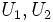$U_1, U_2$ are open subsets of$X$, and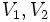$V_1, V_2$ open subsets of$\R^n$, with homeomorphisms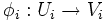$\phi_i: U_i \to V_i$. Then the transition function for these coordinate charts is defined as the map from$\phi_1(U_1 \cap U_2)$ to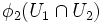$\phi_2(U_1 \cap U_2)$ given by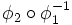$\phi_2 \circ \phi_1^{-1}$ (note that both these maps make sense in the area we are defining).

Intuitively, the transition function is a way to pass from one coordinate chart to another, for those points for which both coordinate charts are valid.